Technical Article

# How to Use Decoupling Capacitor Placement to Reduce Harmonic Distortion

February 12, 2021 by Dr. Steve Arar

## Learn how to reduce second harmonic distortion by following some guidelines for placing decoupling capacitors in your PCB layout.

In a previous article, we discussed that a symmetrical PCB layout is required to reduce the second harmonic distortion.

In this article, we’ll see that, without proper decoupling, we cannot extract the maximum linearity performance from a high-speed op-amp. In addition, we’ll discuss that simply rearranging a decoupling capacitor can affect the distortion performance of a high-speed amplification stage.

### Poor Capacitor Decoupling Can Increase Distortion

The power and ground conductors of a PCB do exhibit some inductance. This inductance can cause issues if we attempt to provide the high-frequency currents of a device directly through the power and ground conductors.

Recall that the voltage drop that appears across an inductor is proportional to the rate of change of current. Hence, at higher frequencies, a relatively larger voltage drop will appear across the power and ground conductors and we won’t be able to deliver a constant supply voltage to the IC.

With a high-speed op-amp, the variations on the supply voltage will be signal-dependent and consequently, the linearity performance will be significantly degraded.

To address this issue, we place decoupling capacitors close to the op-amp supply pins. Acting as a source of charge, decoupling capacitors provide the high-frequency currents and significantly reduce the variations on the supply voltage. The following figure shows the frequency content at the output of the AD9631 op-amp driving a 100Ω load.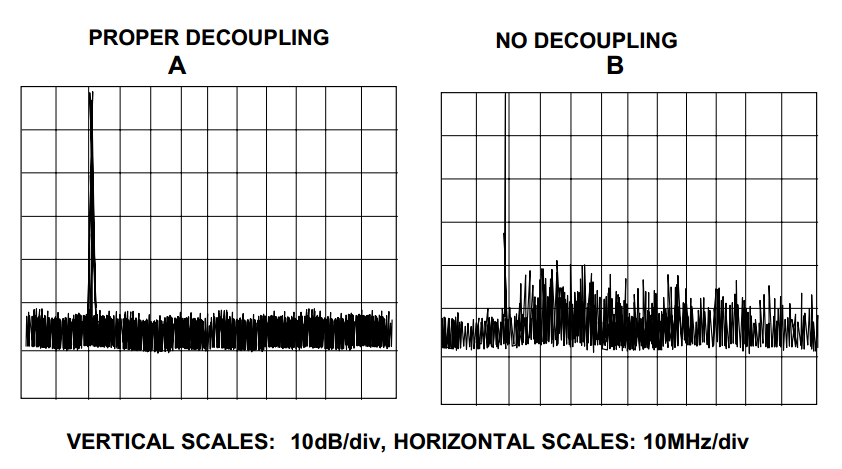##### Figure 1. The spectral output of the AD9631 op amp with proper decoupling (left) and no decoupling (right). Image courtesy of Analog Devices.

As you can see, with proper decoupling, the distortion components are dramatically reduced.

### Keep the Capacitor Ground Terminal Away from the Op-Amp Inputs

PCB layout is a key factor when optimizing the distortion performance of a high-speed board. Consider the layout examples shown below for a non-inverting amplification stage that uses an op-amp in an SOIC package.

In these examples, all components are placed on the top side of the board and only the bypass capacitor of the positive rail (Cbypass1) is on the bottom side. We assume that the board has a dedicated ground plane and the vias, represented by the green circles, connect a trace or pad to this ground plane.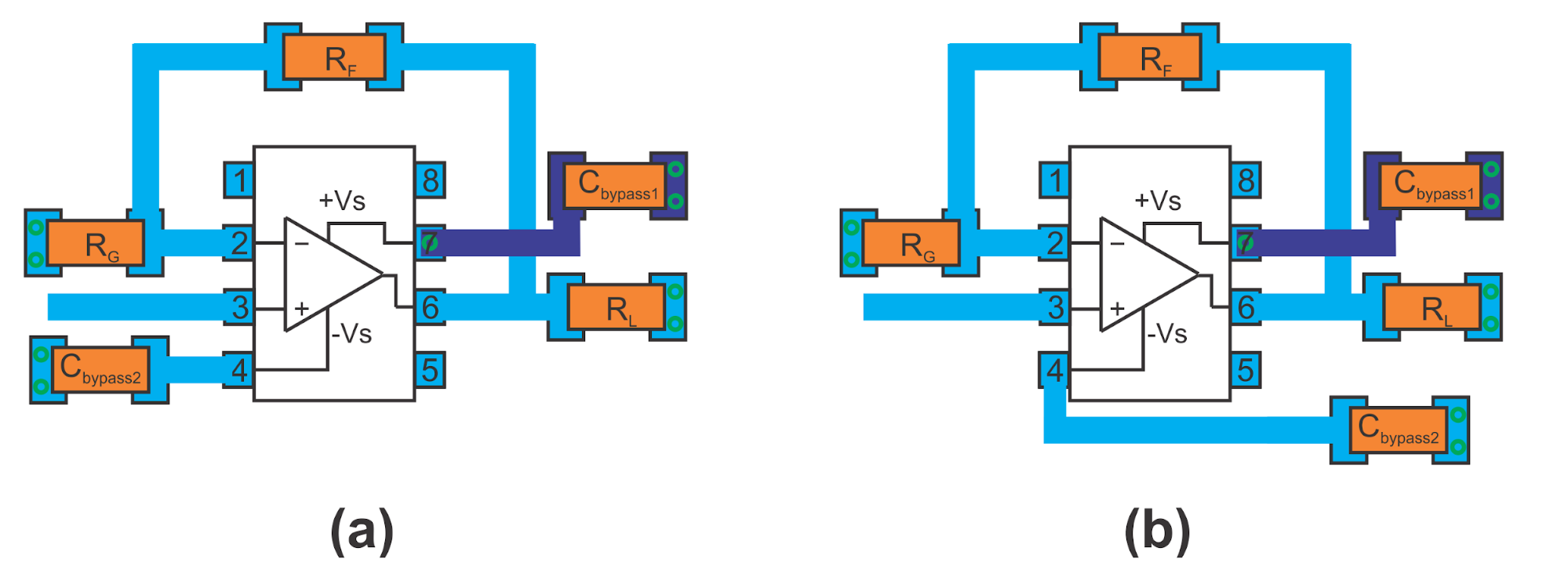##### Figure 2. Two circuit diagrams with differing placement of the bypass capacitor of the negative rail.

As you can see, the two layouts are exactly the same except for the placement of the bypass capacitor of the negative rail (Cbypass2). While the layout on the left places the ground side of Cbypass2 close to the op-amp inputs, the layout on the right side attempts to keep this terminal close to the load and away from the op-amp inputs.

The layout in Figure 2(b) can achieve a better distortion performance.

### Pay Careful Attention to the Return Current Path

To understand why the layout in Figure 2(b) exhibits a lower distortion, consider the return current flowing through the ground plane when the signal applied to the load has a negative polarity, i.e., Cbypass2 is supplying the load current.

When the output signal polarity is negative, the current drawn from the load flows through the top layer trace and the op-amp circuitry as shown by the blue arrows in Figure 3.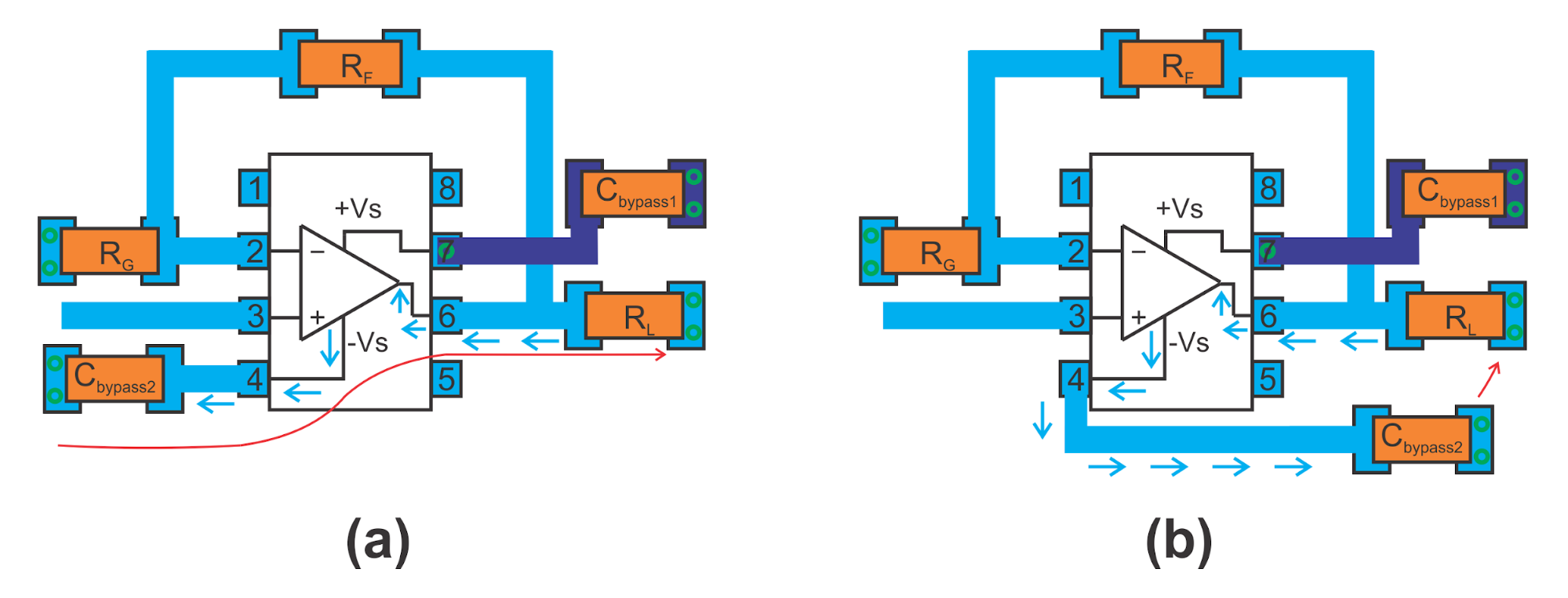##### Figure 3. The same diagrams as in Figure 2, but showing the current flow with blue arrows.

We know that the high-frequency return current flows directly underneath the signal trace to minimize the loop area. Hence, the return current of the layout in Figure 3(a) should follow a path similar to that shown by the red line.

However, it is important to note that, although most of the return current flows directly underneath the signal trace, it still can spread out slightly on the ground plane as shown in Figure 4.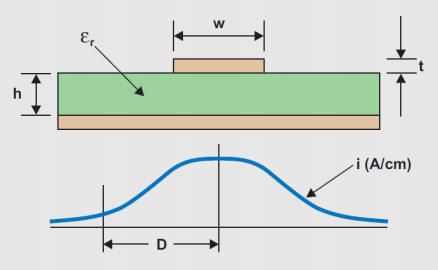##### Figure 4. Distribution of high-frequency return current. Image courtesy of Segera Davies.

Therefore, with the layout in Figure 3(a), the return current can perturb the voltage at the op-amp inputs. The error signal coupled to the op-amp inputs will be signal-dependent and consequently, will cause distortion at the op-amp output. Since the signal-dependent error voltage appears only during one polarity of the output voltage (the negative polarity), it will mainly increase the second harmonic distortion.

What path the return current will choose on the ground plane in Figure 3(b)?

Again, the path directly underneath the signal trace (below the blue arrows) will offer the lowest possible inductance. However, in this case, the ground side of the bypass cap is very close to the ground terminal of the load. Hence, the path shown by the red arrow in 3(a) can offer a very small resistance compared to that of the path of the least inductance. In fact, the return current will choose the path of least impedance (both the path inductance and resistance should be considered).

To determine the exact distribution of the return current, we’ll need simulation tools; however, we can deduce that a portion of the return current will flow around the red arrow and a relatively smaller current will flow underneath the blue arrows. With a relatively smaller current flowing underneath the signal trace, we can expect to have a “quieter” ground below sensitive nodes of the circuit (around the op-amp inputs).

Keeping the ground side of the bypass caps away from the op-amp inputs is an effective technique for reducing harmonic distortion and it is commonly recommended in different technical documents from different chip manufacturers.

### What If the Load Is Far from the Op-Amp Output?

Let's take a look at one more example wherein the load is located at a distance from the op-amp output as shown in Figure 5.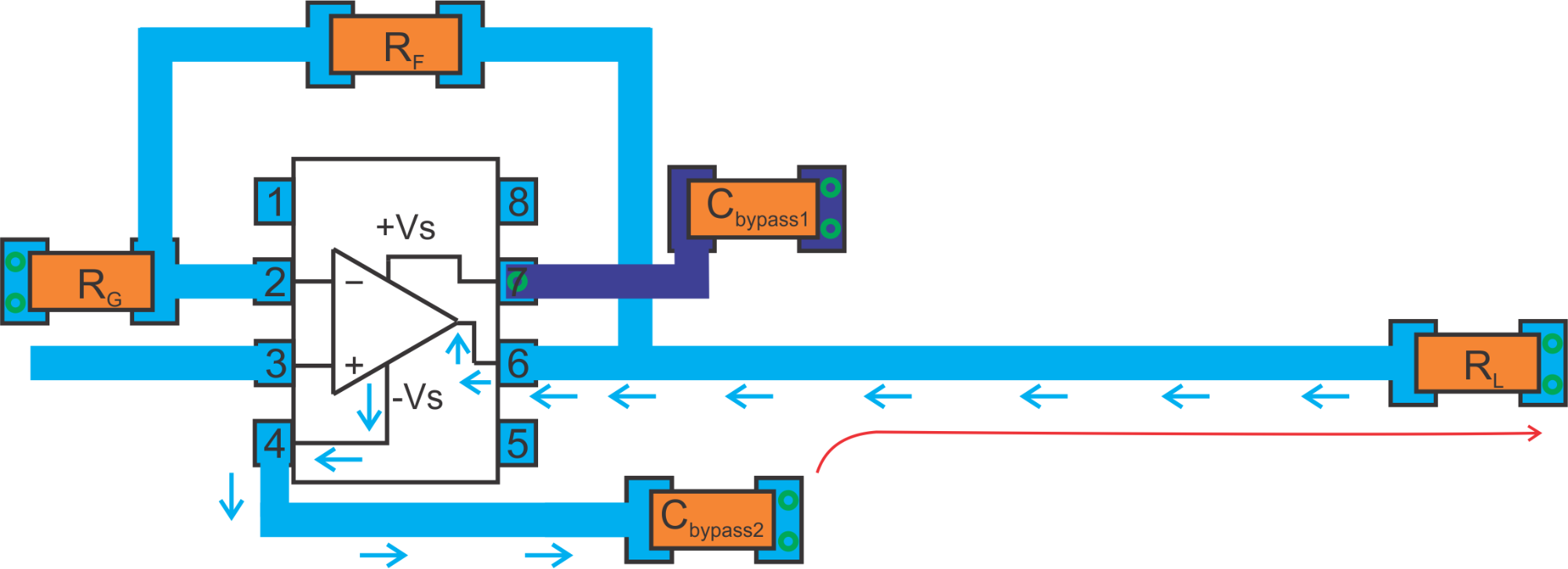##### Figure 5. Our example op-amp circuit but with the load farther from the op-amp output.

Again, we should keep the ground side of the bypass capacitor away from the op-amp inputs. The capacitor should be placed close to the op-amp power supply pin with its ground terminal close to the op-amp output.

A considerable portion of the return current should follow the low-resistance path discussed above leading to the return current path shown by the red line in the following figure.

Proper decoupling is required to extract the maximum linearity performance from a high-speed op-amp. Additionally, the ground side of the bypass capacitor should be placed close to the op-amp out and away from its inputs so that we can have a “quieter” ground under the sensitive nodes of the circuit (around the op-amp inputs).